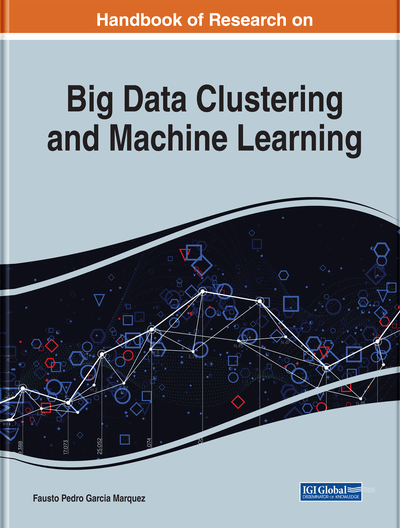# On Foundations of Estimation for Nonparametric Regression With Continuous Optimization

Pakize Taylan (Dicle University, Turkey)
DOI: 10.4018/978-1-7998-0106-1.ch009
Available
\$37.50
No Current Special Offers

## Abstract

The aim of parametric regression models like linear regression and nonlinear regression are to produce a reasonable relationship between response and independent variables based on the assumption of linearity and predetermined nonlinearity in the regression parameters by finite set of parameters. Nonparametric regression techniques are widely-used statistical techniques, and they not only relax the assumption of linearity in the regression parameters, but they also do not need a predetermined functional form as nonlinearity for the relationship between response and independent variables. It is capable of handling higher dimensional problem and sizes of sample than regression that considers parametric models because the data should provide both the model building and the model estimates. For this purpose, firstly, PRSS problems for MARS, ADMs, and CR will be constructed. Secondly, the solution of the generated problems will be obtained with CQP, one of the famous methods of convex optimization, and these solutions will be called CMARS, CADMs, and CKR, respectively.
Chapter Preview
Top

## Introduction

The traditional nonlinear regression model (NRM) fits the model (Bates & Watts, 1988; Seber & Wild, 1989).

,(1) whereis a p-vector for unknown parameters that must be estimated by nonlinear least square estimation method,is a p-vector of explanatory variables of the ith observations, say, andis an suitable function that depends on the explanatory variables and parameters. The functionthat establishes relationship between the average of the response variable y and the explanatory variables, is predetermined. Here,are random errors that describes deviation from the function f and observationsand it is supposed that they are independent and distributed withwhereis constant variance. Nonlinear model is a parametric model and it is supposed that set of unknown parametersis finite. If we have parameters, future estimations of, will be independent of data obtained from observation. For this reasonholds everything you need to know about the data and the complexity of the model will be finite even the amount of data used is infinite. This situation makes NLR model not very flexible. Nonparametric regression (NPR) is established based on the information ensured from the data and it needs larger sizes sample than regression using parametric models like linear and nonlinear regression models because the data should provide both the model building and the model estimates. Nonparametric models suppose that the distribution of data cannot be described by considering such a finite dimensional parameters. But they can usually be desribed by supposing an infinite dimensional. The amount of information thatcan hold about the data can increase as the amount of data increases. This makes NPR more flexible.

The general NPR model (Fox, 2001) is written as

(2) whereis p- vector of explanatory variables for ith realization, but the functionis unspecified unlike NRM. Moreover, the aim of NPR is to produce a model directly for the relationship between response variable and explanatory variables through function, rather than for estimating parameters. Generally, it is supposed implicitly thatis a smooth, continuous function when NPR is used for data analysis. The standart assumption for NPR is that, as in NRM.

## Complete Chapter List

Search this Book:
Reset